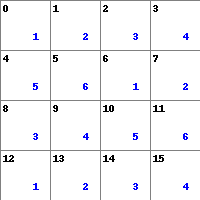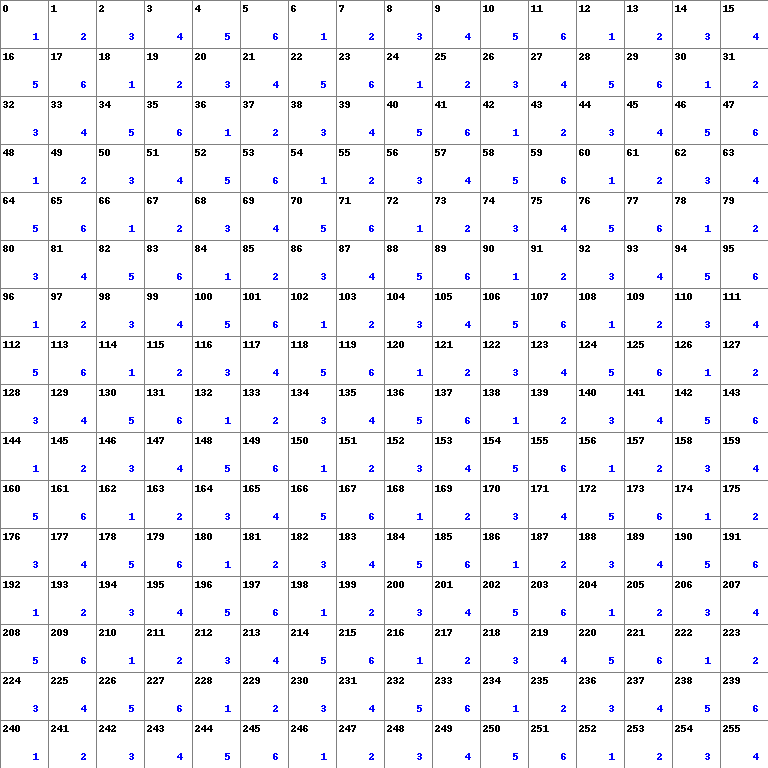# Pseudo Random Numbers in a Range and Modulo BiasIt is sometimes necessary to obtain a pseudo-random number from a specific range. For example:

• Simulating a fair die roll, where the value should be between 1 and 6 inclusive
• Randomly selecting a word from a word list - for example, generating a secure passphrase

This can be achieved by using modular arithmetic whereby numbers are limited to a certain range, wrapping around when the range is exceeded.

## Selecting From a Range

Suppose you have a random number generator that generates numbers with a sufficient degree of randomness in the range [0, 16) - i.e. 16 integers, from 0 to 15 inclusive.

You need a random number in the range [1, 6].

To achieve this, you could reduce the range of the random number by modular division:

• Determine an appropriate modulus, m such that m = upper bound - lower bound + 1 (in this case, 6 - 1 + 1 = 6)
• Compute the remainder (mod m) of the random number returned
• Add this to the lower bound (in this case, 1) to get a random number in the required range

The diagram below shows each possible random number in our 16 number range in black, with the remainder mod 6 shown in blue:From this diagram, you can see that the probability of returning a number of 1 to 6 is NOT evenly distributed. You can easily see probabilities in this case by simply counting how many times they occur and dividing by the total number of possible values:

Mod 6 Value + 1, Random Number 0-15 Probability
1 3/16
2 3/16
3 3/16
4 3/16
5 2/16
6 2/16

In this case the values 5 and 6 have a (slightly) lower probability of being returned, even if the original random number (between 0 and 15) is truly random. This effect is known as modulo bias.

## Random Bytes

Modern computers operate effectively at the level of the byte (8 bits) - under Linux, you might get a pseudo-random number by fetching a byte from the `/dev/urandom` or `/dev/random` devices - these are basically the Linux kernel API for accessing randomness.

This random byte represents a decimal number between 0 (an empty byte) and 255 (wherein all bits are set).

To continue with the fair die analogy, consider how we might return a number in the range [1, 6] for all possible values of a single byte:This time the absolute difference in probabilities is smaller, because the possible range for our original random number is greater - there are 256 possibilities compared to 16:

Mod 6 Value + 1, Random Number 0-255 Probability
1 43/256
2 43/256
3 43/256
4 43/256
5 42/256
6 42/256

## Correcting for Modulo Bias

We can correct modulo bias by discarding any random number in excess of the maximum exact multiple of our modulus.

In other words:

• Determine the modulus m such that we return numbers in the required range [lower bound, upper bound]: upper bound - lower bound + lower bound
• Take the number of possible random values - this will be the maximum possible value + 1 to account for the possibility of zero
• Determine the remainder of dividing the number of possible random numbers by m
• Subtract this excess from the maximum possible random number

The result is a threshold above which we discard random numbers. If the random number exceeds this threshold, we re-roll our random number generator until we return a value that is within the range. This ensures that the final results are evenly distributed.

Taking the 16 value example above, we would discard random values greater than or equal to 12:

• 16 (mod 6) ≡ 4
• 16 - 4 = 12
• If the random integer is > 11 we should reject it

Doing the same thing for the one-byte example, 251 is our threshold above which we should reject values.

## Example: Python

This example uses the range-restricted random number to pseudo-randomly select a word from a wordlist:

``````#!/usr/bin/env python3
import sys
import os
from subprocess import check_output

class RandomInRange:
"""
Class that provides a pseudo-random number within a given range,
corrected for modulo bias.
"""
def __init__(self, lower, upper):
self.lower = lower
self.upper = upper
self.random_src_path = '/dev/urandom'
self.set_parameters()

def set_parameters(self):
max_bytes = {
1: (1 <<  8) - 1, # Max required random number is 2⁸ - 1
2: (1 << 16) - 1, # Max required random number is 2¹⁶ - 1
3: (1 << 24) - 1, # Max required random number is 2²⁴ - 1
4: (1 << 32) - 1  # Max required random number is 2³² - 1
}

# Compute modulus m that will be used to restrict range
self.m = self.upper - self.lower + 1
try:
assert(self.m < max_bytes), "Max value exceeded."
except AssertionError as error:
print(error)
print("The range magnitude {} is too large.".format(self.m))
sys.exit(1)

# Determine number of pseudo random bytes needed and the related max random value
for i in range(1, 4):
if self.m <= max_bytes[i]:
self.n_bytes = i
self.max_random = max_bytes[i]
break

self.excess = (self.max_random % self.m) + 1
self.max_allowed = self.max_random - self.excess

def random_number(self):
while True:
data = os.urandom(self.n_bytes)
data = int.from_bytes(data, "big")
if data < self.max_allowed:
break
return (data % self.m) + self.lower

class RandomWords:
"""
Class that builds a list of randomly-selected words.
"""
def __init__(self, n):
# Number of words
self.n = n
# Initialise an empty list for the randomly selected words
self.random_words = list()
# The word list to select from (Ubuntu/Debian)
self.words = '/usr/share/dict/cracklib-small'
# Number of lines in the wordlist file
self.keyspace = sum(1 for line in open(self.words))
# Initialise a RandomInRange object
self.r = RandomInRange(1, self.keyspace)
self.make_list()

def make_list(self, n=None):
"""
Randomly select words from a wordlist and append them to a list.
Uses a modular range-reduced random number to make the selection
without modulo bias.
"""
n = n if n else self.n
for i in range(0, n):
r_num = self.r.random_number()
self.random_words.append(self.get_one_line(r_num).decode('utf-8').rstrip())

def get_one_line(self, line_number):
"""
sed is a reasonable way to get a line from a file indexed by line number.
"""
return check_output([
"sed",
"-n",
"%sp" % line_number,
self.words
])

def print_words(self):
for w in self.random_words:
print(w)

def main():
r = RandomWords(24)
r.print_words()

if __name__ == '__main__':
main()
``````

## Example: Bash

``````#!/bin/bash
#
#
# you may not use this file except in compliance with the License.
# You may obtain a copy of the License at
#
#
# Random Number Within a Specific Range
# -------------------------------------
# To obtain a random number within a particular (inclusive) range within a Bash script:
# * Source this file
# * Run `random_number_in_range <lower bound> <upper bound>`
# * The `\$random_number` variable in your calling script will have an
# appropriate random number
#
# If you run `random_number_in_range` with no parameters, you will be prompted to enter
# a lower and upper value.
# -----------------------------------------------------------------------------------------------------------
RANDOM_SOURCE="/dev/urandom"
ONE_BYTE_MAX=\$(( (1 « 8) - 1 ))
TWO_BYTE_MAX=\$(( 1 « 16 - 1 ))
THREE_BYTE_MAX=\$(( (1 « 24) - 1 ))

function set_n_bytes {
lower=\$1
upper=\$2
m=\$((\$upper - \$lower + 1))
if (( \$m < \$ONE_BYTE_MAX )); then
n_random_bytes=1
max_random=\$ONE_BYTE_MAX
elif (( \$m > \$ONE_BYTE_MAX && \$m < \$TWO_BYTE_MAX )); then
n_random_bytes=2
max_random=\$TWO_BYTE_MAX
elif (( \$m > \$TWO_BYTE_MAX && \$m < \$THREE_BYTE_MAX )); then
n_random_bytes=3
max_random=\$THREE_BYTE_MAX
elif (( \$m >= \$THREE_BYTE_MAX )); then
echo "Too big for this script"
exit 1
fi

mod=\$(( \$upper - \$lower + 1))
excess=\$(( (\$max_random % \$mod) + 1 ))
max_allowed=\$(( \$max_random - \$excess ))
}

function random_in_range {
if [[ \$excess != 0 ]]; then
while (( 1 )); do
random_number=\$(od -N\${n_random_bytes} -An -i \${RANDOM_SOURCE})
if (( \$random_number <= \$max_allowed )); then
break
fi
done
else
random_number=\$(od -N\${n_random_bytes} -An -i \${RANDOM_SOURCE})
fi
random_number=\$(( (\$random_number % \$mod) + \$lower ))
}

function set_inputs {
if [[ \$# -eq 0 ]]; then
echo "Enter lower (inclusive) bound:"
echo "Enter upper (inclusive) bound:"
else
lower=\$1
upper=\$2
fi
set_n_bytes \$lower \$upper
}

function random_number_in_range {
set_inputs \$1 \$2
random_in_range
}

function test_run {
. tests
set_inputs 1 6
test_diceroll
set_inputs 1 4
test_4_sided_diceroll
}

[[ \$1 == "test" ]] && test_run
``````

NOTE: If you copy and paste the above code it will error - I used digraphs for the left-shift operators because markdown syntax highlighting thinks `<<` should start a heredoc, even inside a `\$(( ))` block.

If you want a copy of this script you can get it here.

## A Note on Randomness

Remember that your source of “Randomness” is very important. For most purposes, we can use Pseudo random number generators such as `/dev/urandom` and `/dev/random` on Linux.

Anyone who considers arithmetical methods of producing random digits is, of course, in a state of sin. For, as has been pointed out several times, there is no such thing as a random number — there are only methods to produce random numbers, and a strict arithmetic procedure of course is not such a method.

John Von Neumann

Because computers are deterministic, they can only ever produce pseudo-randomness.

• GitHub repo for this post with examples using Bash and Python to generate randomly selected words
• Randomness in C++ - I included a “diceroll” example here that simulates a diceroll using C++ and the Linux kernel randomness API